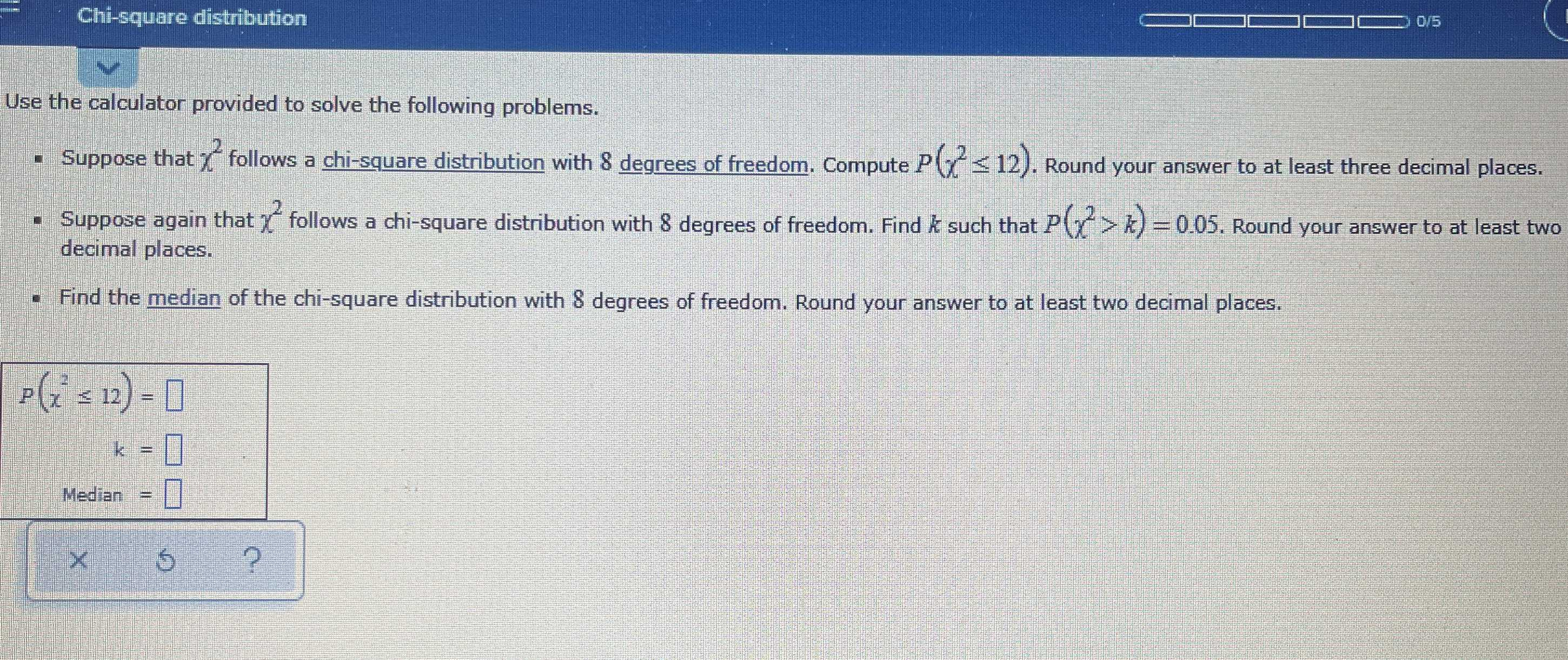### Still have math questions?

Algebra
QuestionUse the calculator provided to solve the following problems.

- Suppose that $$\chi ^ { 2 }$$ follows a chi-square distribution with $$8$$ degrees of freedom. Compute $$P ( \chi ^ { 2 } \leq 12 )$$ . Round your answer to at least three decimal places.

Suppose again that $$\chi ^ { 2 }$$ follows a chi-square distribution with $$8$$ degrees of freedom. Find $$k$$ such that $$P ( x ^ { 2 } > k ) = 0.05$$ . Round your answer to at least two decimal places.

- Find the median of the chi-square distribution with $$8$$ degrees of freedom. Round your answer to at least two decimal places.